# Numerical Solution of Resonant Layer Equations

Let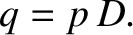(6.27)

Equations (6.5)–(6.7) yield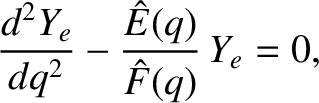(6.28)

where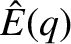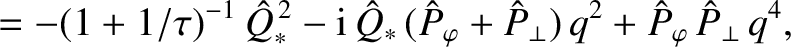(6.29)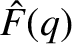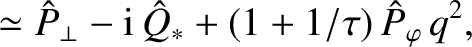(6.30)

and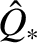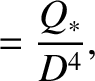(6.31)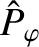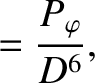(6.32)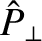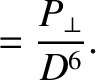(6.33)

Equation (6.28) must be solved subject to the constraint that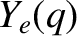is bounded as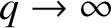, and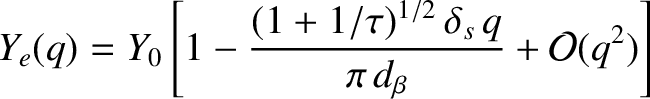(6.34)

as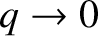. Here, use has been made of Equations (6.8), (6.26), and (6.27). As is easily demonstrated (see Section 5.14), the solution of Equation (6.28) that is bounded asis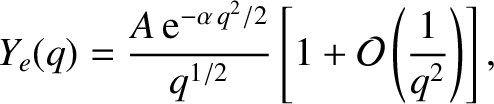(6.35)

where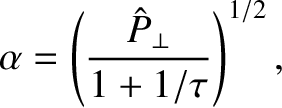(6.36)

and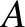is an arbitrary constant.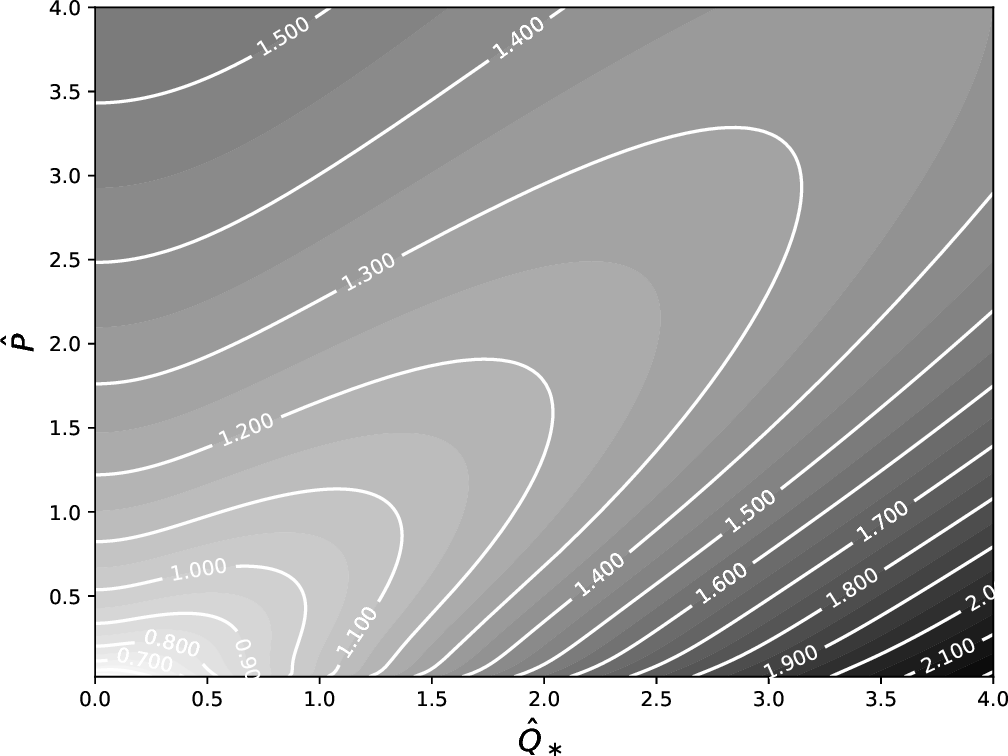Let us again (see Section 5.14) make the Riccati transformation [2,5]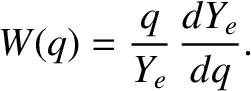(6.37)

Equation (6.28) yields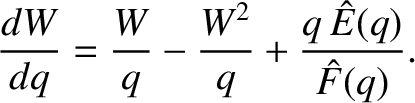(6.38)

According to Equation (6.34), the small-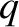behavior of the solution to the previous equation is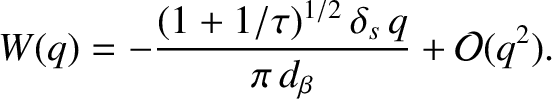(6.39)

Likewise, according to Equation (6.35), the large-behavior of the solution is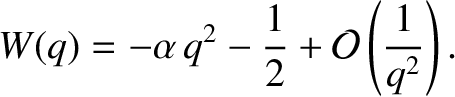(6.40)

Equation (6.38) is conveniently solved numerically by launching a solution of the form (6.40) at large, and then integrating backward to small. It follows from Equation (6.39) that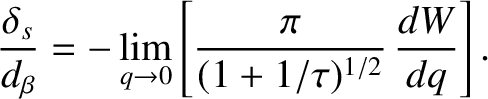(6.41)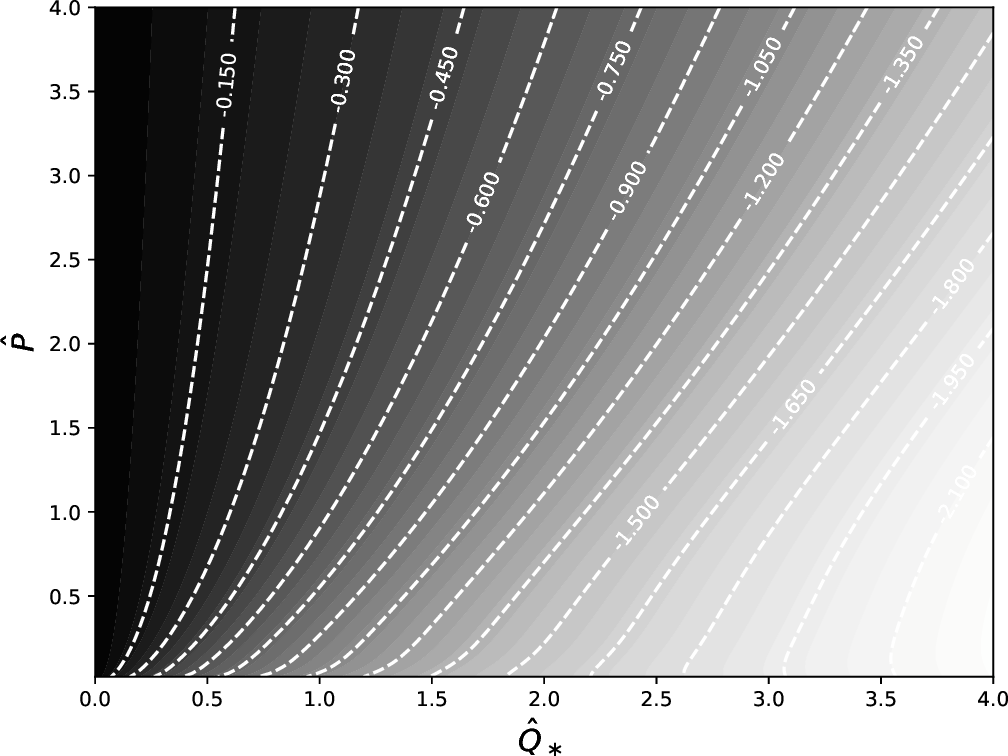Table 6.2 gives estimates for the normalized resonant layer parameters,,, and, that appear in Equations (6.28)–(6.30), in a low-field and a high-field tokamak fusion reactor. These estimates are made using the data shown in Table 6.1. Table 6.2 also gives estimates for the linear layer thicknesses and tearing mode growth-rates in such reactors. These estimates are obtained via numerical solution of the resonant layer equation. It can be seen that the typical radial thickness of a linear tearing layer in a tokamak fusion reactor is only a few millimeters. Furthermore, linear tearing modes in tokamak fusion reactors grow on timescales that typically lie between a tenth of a second and a second [assuming that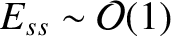.] Finally, the real frequencies of such modes, in a frame of reference that co-rotates with the electron fluid at the resonant surface, (i.e., the imaginary components of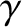) are very much smaller (by a factor of order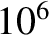) than a typical diamagnetic frequency. (See Table 6.1.) In other words, linear tearing modes do indeed co-rotate with the electron fluid at the resonant surface to a very high degree of fidelity.

Figures 6.2 and 6.3 show values of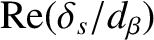and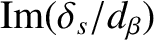, respectively, evaluated numerically as functions ofand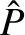. In producing these figures, it is assumed that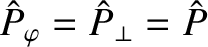. The fact that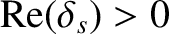for all values ofandconfirms that tearing modes are linearly unstable for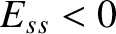, and stable otherwise . [See Equation (6.25).] It is clear that the layer width increases with increasing normalized diamagnetic frequency,, and also with increasing normalized magnetic Prandtl number,.